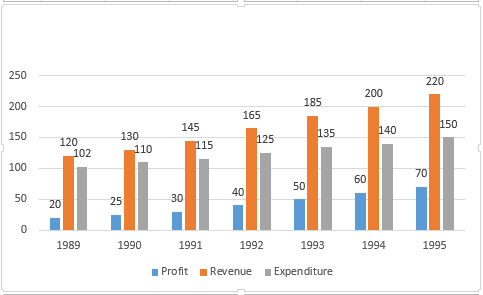### CAT 1996 Question Paper Question 47

Instructions

Answer the questions based on the following information.Question 47

# The expenditure for the 7 years together form what per cent of the revenues during the same period?

Solution

Total expenditure = 102 + 110 + 115 + 125 + 135 + 140 + 150 = 877
Total revenue = 120 + 130 + 145 + 165 + 185 + 200 + 220 = 1165
%expenditure to revenue = $$\frac{877}{1165} \times 100$$ = 75 (nearly)